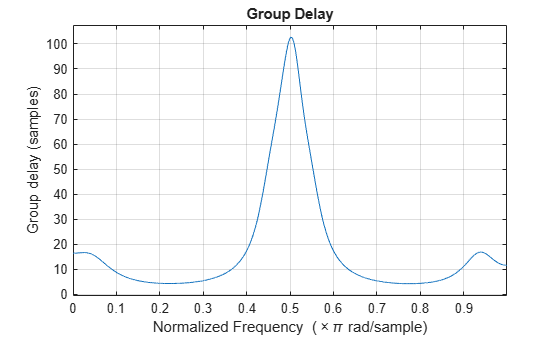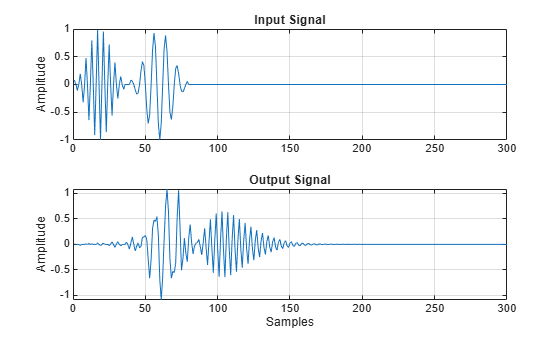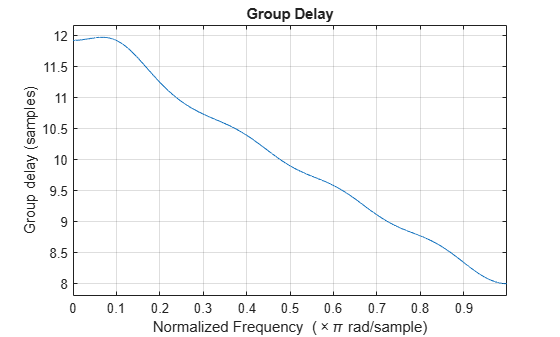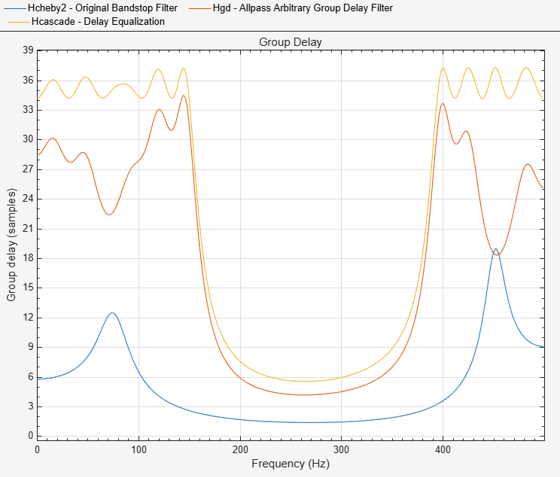# fdesign.arbgrpdelay

Arbitrary group delay filter specification object

## Syntax

``specObj = fdesign.arbgrpdelay``
``specObj = fdesign.arbgrpdelay(spec)``
``specObj = fdesign.arbgrpdelay(spec,value1,...,valueN)``
``specObj = fdesign.arbgrpdelay(N,F,Gd)``
``specObj = fdesign.arbgrpdelay(___,Fs)``

## Description

Arbitrary group delay filters are allpass filters that you can use to correct the phase distortion introduced by other filters. The `fdesign.arbgrpdelay` function uses an iterative least p-th norm optimization procedure to minimize the phase response error .

The `fdesign.arbgrpdelay` function returns a filter design specification object containing filter specifications, such as filter order, number of bands, frequency vector, and group delay response. You then use the `design` function to design the filter from the filter design specifications object.

For more control options, see Filter Design Procedure. For a complete workflow, see Design a Filter in Fdesign — Process Overview.

````specObj = fdesign.arbgrpdelay` creates an allpass arbitrary group delay filter design specification object with the filter order of 10, frequency vector of [0 0.1 1], and group delay response vector of [2 3 1].```
````specObj = fdesign.arbgrpdelay(spec)` creates a filter design specification object with the specification property `spec`. For more information on the specifications that the function supports, see spec.```

example

````specObj = fdesign.arbgrpdelay(spec,value1,...,valueN)` creates a filter design specification object with the specification values `value1,...,valueN`. Set the specification options in the expression `spec`. After the expression, specify a value for each option.```

example

````specObj = fdesign.arbgrpdelay(N,F,Gd)` creates a filter design specification object with the filter order, frequency vector, and group delay response vector specified in `N`, `F`, and `Gd`, respectively. For more information on the filter order, frequency vector, and group delay vector inputs, see spec.```

example

````specObj = fdesign.arbgrpdelay(___,Fs)` specifies the sample rate in Hz. If you specify a sample rate, the group delay response values in the filter design specification object are in seconds. If you do not specify a sample rate, the function normalizes all frequencies and the group delay response values are in samples.```

## Examples

collapse all

Create a signal consisting of two discrete-time windowed sinusoids (wave packets) with disjoint time support to illustrate frequency dispersion. One discrete-time sinusoid has a frequency of $\pi$/2 radians/sample and the other has a frequency of $\pi$/4 radians/sample. There are nine periods of the sinusoid with the higher frequency that precede five periods of the signal with the lower frequency.

Create the signal.

```x = zeros(300,1); x(1:36) = cos(pi/2*(0:35)).*hamming(36)'; x(40:40+39) = cos(pi/4*(0:39)).*hamming(40)';```

Create an arbitrary group delay filter that delays the wave packet with the higher frequency by approximately 100 samples.

```N = 18; f = 0:.1:1; gd = ones(size(f));```

Delay $\pi$/2 radians/sample by 100 samples.

```gd(6) = 100; d = fdesign.arbgrpdelay(N,f,gd); Hd = design(d,'iirlpnorm',MaxPoleRadius=0.9,SystemObject=true);```

Visualize the group delay.

`fvtool(Hd,analysis='grpdelay');`Filter the input signal with the arbitrary group delay filter and plot the frequency dispersion. The high-frequency wave packet, which initially preceded the low-frequency wave packet, now occurs later because of the nonconstant group delay.

```y = Hd(x); subplot(211) plot(x); title('Input Signal'); grid on; ylabel('Amplitude'); subplot(212); plot(y); title('Output Signal'); grid on; xlabel('Samples'); ylabel('Amplitude');```Design an allpass filter with an arbitrary group delay using the `fdesign.arbgrpdelay` and the `design` functions. Pass `iirlpnorm` as the design method.

``` N = 10; f = [0 0.02 0.04 0.06 0.08 0.1 0.25 0.5 0.75 1]; g = [5 5 5 5 5 5 4 3 2 1]; w = [2 2 2 2 2 2 1 1 1 1]; hgd = fdesign.arbgrpdelay(N,f,g); Hgd = design(hgd,'iirlpnorm',Weights=w,MaxPoleRadius=0.95,... SystemObject=true)```
```Hgd = dsp.SOSFilter with properties: Structure: 'Direct form II' CoefficientSource: 'Property' Numerator: [5x3 double] Denominator: [5x3 double] HasScaleValues: true ScaleValues: [0.2292 1 1 1 1 1.3458] Show all properties ```
` fvtool(Hgd,Analysis='grpdelay') ;`Perform group delay equalization of a bandstop Chebyshev filter operating with a sample rate of 1 kHz.

```Fs = 1e3; Hcheby2 = design(fdesign.bandstop('N,Fst1,Fst2,Ast',10,150,400,60,Fs),'cheby2',... SystemObject=true); f1 = 0.0:0.5:150; % Hz g1 = grpdelay(Hcheby2,f1,Fs).'/Fs; % seconds f2 = 400:0.5:500; % Hz g2 = grpdelay(Hcheby2,f2,Fs).'/Fs; % seconds maxg = max([g1 g2]);```

Design an arbitrary group delay allpass filter to equalize the group delay of the bandstop filter. Use an 18 order multiband design and specify two bands.

```hgd = fdesign.arbgrpdelay('N,B,F,Gd',18,2,f1,maxg-g1,f2,maxg-g2,Fs); Hgd = design(hgd,'iirlpnorm',MaxPoleRadius=0.95,SystemObject=true); Hcascade = cascade(Hcheby2,Hgd); hft = fvtool(Hcheby2,Hgd,Hcascade,Analysis='grpdelay',Fs=Fs); legend(hft,'Original Bandstop Filter','Allpass Arbitrary Group Delay Filter',... 'Delay Equalization', 'Location','North');```## Input Arguments

collapse all

Filter specification, specified as one of these character vectors:

• `'N,F,Gd'`

• `'N,B,F,Gd'`

This table describes the options available in each expression.

Specification OptionDescription
`N`Filter order (must be even)
`F`Frequency vector
`Gd`Group delay response vector
`B`Number of frequency bands

The design methods that you can use to design the filter depend on the specification expression. You can obtain these methods using the `designmethods` function. This table lists the specification expressions supported by the `fdesign.arbgrpdelay` function and the corresponding design method.

Specification ExpressionSupported Design MethodFilter Description
`'N,F,Gd'``iirlpnorm`

Least P-norm optimal IIR filter

`'N,B,F,Gd'``iirlpnorm`

Least P-norm optimal IIR filter

To design the filter, call the `design` function with one of the design methods as an input. For more details on the procedure, see Filter Design Procedure. For an example, see Design an Allpass Filter With an Arbitrary Group Delay.

Specification values, specified as a comma-separated list of values. Specify a value for each option in `spec` in the same order as the options in the specification expression.

Example: ```specObj = fdesign.arbgrpdelay('N,F,Gd',N,F,Gd)```

The arguments below describe more details for each option in the expression.

Filter order, specified as an even positive integer. The numerator and denominator orders are both equal to `N`. For more information on why the numerator and denominator filter orders are equal and even in the `fdesign.arbgrpdelay` function, see Allpass Systems.

Data Types: `single` | `double` | `int8` | `int16` | `int32` | `int64` | `uint8` | `uint16` | `uint32` | `uint64`

Frequency vector for the group delay specifications, specified as a row vector. The elements of the frequency vector must increase monotonically.

If you do not specify a sample rate `Fs`, the function normalizes the frequencies. For a single-band design, the first element of the normalized frequency vector must be 0 and the last element must be 1. These correspond to 0 and π radians/sample, respectively. For multiband designs, the union of the frequency vectors must range between [0, 1].

If you specify a sample rate `Fs`, the first element of the frequency vector in a single-band design must be 0. The last element must be the Nyquist frequency `Fs`/2. For multiband designs, the union of the frequency vectors must range between [0, `Fs`/2].

Data Types: `double`

Group delay vector, specified as a row vector with nonnegative elements and equal in length to the frequency vector `F`. The elements of `Gd` specify the nonnegative group delay at the corresponding element of the frequency vector `F`.

If you do not specify a sample rate `Fs` in Hz, the group delay values are in samples. If you specify a sample rate, the group delay values are in seconds.

Data Types: `double`

Number of frequency bands, specified as a positive integer. To use this argument, you must specify a frequency vector `F` and a group delay vector `Gd` for each band. The union of the frequency vectors must range between [0, 1] in normalized frequency or [0, `Fs`/2] when you specify a sample rate. The elements in the union of the frequency bands must be monotonically increasing.

Example:

```filtorder = 14; freqband1 = [0 0.1 0.4]; grpdelay1 = [1 2 3]; freqband2 = [0.5 0.8 1]; grpdelay2 = [3 2 1]; D = fdesign.arbgrpdelay('N,B,F,Gd',filtorder,2,freqband1,grpdelay1,freqband2,grpdelay2);```

Data Types: `single` | `double` | `int8` | `int16` | `int32` | `int64` | `uint8` | `uint16` | `uint32` | `uint64`

Sample rate, specified as a positive scalar. Specify this argument after all the other input arguments. Specifying a sample rate forces the group delay units to be in seconds. If you specify a sample rate, the first element of the frequency vector must be 0 and the last element must be the Nyquist frequency `Fs`/2.

Data Types: `single` | `double` | `int8` | `int16` | `int32` | `int64` | `uint8` | `uint16` | `uint32` | `uint64`

## Output Arguments

collapse all

Allpass arbitrary group delay filter design specification object, returned as a `arbgrpdelay` object. The object properties depend on the `spec` input argument.

The filter design specification object can contain one or more of these properties:

• `Specification`

• `NormalizedFrequency`

• `FilterOrder`

• `Frequencies`

• `GroupDelay`

• `NBands` –– Appears if the `Specification` property is set to `'N,B,F,Gd'`.

Use the `normalizefreq` function to change the `NormalizedFrequency` property after constructing the specification object.

collapse all

### Group Delay in Discrete-Time Filter Design

The frequency response of a rational discrete-time filter is:

`$H\left({e}^{j\omega }\right)=\frac{B\left({e}^{j\omega }\right)}{A\left({e}^{j\omega }\right)}$`

The argument of the frequency response as a function of the angle, ω, is referred to as the phase response.

The negative of the first derivative of the argument with respect to ω is the group delay.

`$\tau \left(\omega \right)=-\frac{d}{d\omega }Arg\left(H\left({e}^{j\omega }\right)\right)$`

Systems with nonlinear phase responses have nonconstant group delay, which causes the frequency components of the signal to disperse. You may not want this phase distortion even if the magnitude distortion introduced by the filter produces the desired effect. See Design Arbitrary Group Delay Filter for an illustration of frequency dispersion resulting from nonconstant group delay.

In these cases, you can cascade the frequency-selective filter with an allpass filter that compensates for the group delay. This process is often referred to as delay equalization.

### Allpass Systems

The general form of an allpass system function with a real-valued impulse response is:

`${H}_{ap}\left(z\right)=\prod _{k=1}^{M}\frac{{z}^{-1}-{d}_{k}}{1-{d}_{k}{z}^{-1}}\prod _{k=1}^{N}\frac{\left({z}^{-1}-{c}_{k}\right)\left({z}^{-1}-{c}_{k}^{*}\right)}{\left(1-{c}_{k}{z}^{-1}\right)\left(1-{c}_{k}^{*}{z}^{-1}\right)}$`

where the dk denote the real-valued poles and the ck denote the complex-valued poles, which occur in conjugate pairs.

This equation shows that allpass systems with real-valued impulse responses have 2N+M zeros and poles. The poles and zeros occur in pairs with reciprocal magnitudes. The filter order is always the same for the numerator and denominator.

Because the `fdesign.arbgrpdelay` function uses robust second-order section (biquad) filter structures to implement the allpass arbitrary group delay filter, the filter order must be even.

## Tips

If your arbitrary group delay design produces the ```Poorly conditioned Hessian matrix``` error, perform one of the following or both.

## Algorithms

The `fdesign.arbgrpdelay` function uses a least p-th norm iterative optimization described in .

 Antoniou, A. Digital Signal Processing: Signals, Systems, and Filters., New York:McGraw-Hill, 2006, pp. 719–771.